# EE GATE 2017 PAPER 01 - Online Test

Q1.
The input voltage VDC of the buck-boost converter shown below varies from 32 V to 72 V. Assume that all components are ideal, inductor current is continuous, and output voltage is ripple free. The range of duty ratio D of the converter for which the magnitude of the steady state output voltage remains constant at 48 V is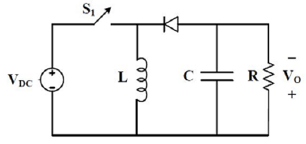Explaination / Solution:Q2. The Boolean expression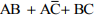simplifies to
Explaination / Solution:Q3. The output expression for the Karnaugh map shown below is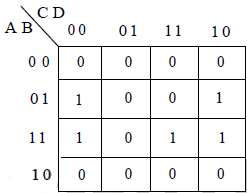Explaination / Solution: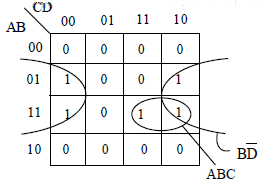Q4. The logical gate implemented using the circuit shown below where. V1 and V2 are inputs (with 0 V as digital 0 and 5 V as digital 1) and Vout is the output isExplaination / Solution: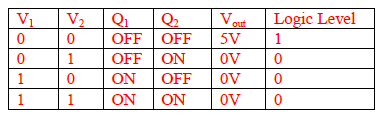So, this logic level o/p is showing the functionality of NOR-gate.

Q5. Find the smallest number y such that y×162 is a perfect cube.
Explaination / Solution:

Factorization of 162 is 2×3×3×3×3 y×162 is a perfect cube y×2×3×3×3×3 Perfect cube For perfect cube 2's & 3's are two more required each.

Q6. Six people are seated around a circular table. There are at least two men and two women. There are at least three right-handed persons. Every woman has a left-handed person to her immediate right. None of the women are right-handed. The number of women at the table is
Explaination / Solution:

Out of six people, 3 place definitely occupied by right handed people as atleast 2 women are there so these two will sit adjacently. Now as only one seat is left it will be occupied by a left handed man because on right side of this seat is sitting an right handed man.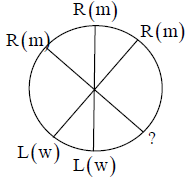Therefore, answer should be 2 women.

Q7.
A contour line joins locations having the same height above the mean sea level. The following is a contour plot of a geographical region. Contour lines are shown at 25m intervals in this plot. If in a flood, the water level rises to 525m. Which of the villages P,Q,R,S,T get submerged?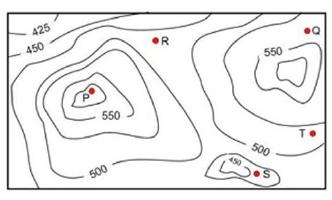Explaination / Solution:

The given contour is a hill station, the peak point of this hill station is P, it is under a contour of 550. At floods, the water level is 525m. So the village of R, S and T are under a contour of 500. Therefore these villages are submerged.

Q8. A source is supplying a load through a 2-phase, 3-wire transmission system as shown in figure below. The instantaneous voltage and current in phase-a are Van = 220sin(100πt)V and ia = 10sin(100πt)A, respectively. Similarly for phase-b the instantaneous voltage and current are Vbn = 220cos(100πt)V and ib = 10cos(100πt)A, respectively.The total instantaneous power flowing form the source to the load is
Explaination / Solution: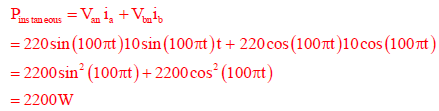Q9. A 4 pole induction machine is working as an induction generator. The generator supply frequency is 60 Hz. The rotor current frequency is 5 Hz. The mechanical speed of the rotor in RPM is
Explaination / Solution: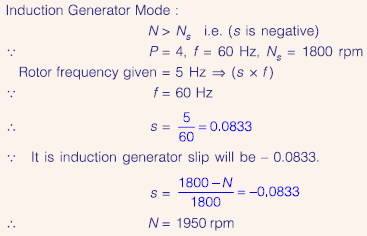Q10. Arun, Gulab, Neel and Shweta must choose one shirt each from a pile of four shirts coloured red, pink, blue and white respectively. Arun dislikes the colour red and Shweta dislikes the colour white, Gulab and Neel like all the colours. In how many different ways can they choose the shirts so that no one has a shirt with a colour he or she dislikes?
Explaination / Solution:

As there are 4 people A,G,N,S and 4 colours so without any restriction total ways have to be 4×4 = 16 Now, Arun⟶dislikes Red and Shweta⟶dislikes white So 16-2=14 ways